# Use the figure at the right. 1. Name the vertex of SOLUTION: U 2

## Transcription

Use the figure at the right. 1. Name the vertex of SOLUTION: U 2
```∠CFD is an acute angle. The measure of ∠CFD is
40.
1-4 Angle Measure
Use the figure at the right.
6. SOLUTION: 1. Name the vertex of
∠ AFD is an obtuse angle. The measure of ∠AFD
is 150.
SOLUTION: U
7. 2. Name the sides of
SOLUTION: SOLUTION: 3. What is another name for
SOLUTION: XYU, UYX
∠BFC is a right angle. The measure of ∠BFC is
90.
4. What is another name for
SOLUTION: 1, YXU
Copy the diagram shown, and extend each ray.
Classify each angle as right, acute, or obtuse.
Then use a protractor to measure the angle to
the nearest degree.
8. SOLUTION: ∠ AFB is an acute angle. The measure of ∠AFB is
25
5. SOLUTION: ∠CFD is an acute angle. The measure of ∠CFD is
40.
ALGEBRA In the figure,
opposite rays,
bisects 9. If
and and are find SOLUTION: 6. eSolutions Manual - Powered by Cognero
SOLUTION: definition of bisecting rays, .Because of the
Page 1
.
∠ AFBMeasure
1-4 Angle
is an acute angle. The measure of ∠AFB is
25
ALGEBRA In the figure,
opposite rays,
bisects and 10. If
are and find SOLUTION: By the Angle Addition
Postulate,
.
bisects Since
9. If
and find .
Thus, .
SOLUTION: .Because of the
.
definition of bisecting rays, Find an expression for
To find 8x – 6, substitute the value 13 for x.
To find 7x – 5, substitute this in for x.
10. If
and find SOLUTION: By the Angle Addition
Postulate,
.
11. CCSS PRECISION A miter cut is used to build
picture frames with corners that meet at right angles.
a. José miters the ends of some wood for a picture frame at congruent angles. What is the degree
measure of his cut? Explain and classify the angle.
b. What does the joint represent in relation to the
angle formed by the two pieces?
bisects Since
Thus, .
.
SOLUTION: a. 45; When joined together, the angles form a right
angle, which measures 90. If the two angles that
form this right angle are congruent, then the measure
of each angle is 90 ÷ 2 or 45. The angle of the cut is an acute angle.
b. The joint is the angle bisector of the frame angle.
Find an expression for
eSolutions Manual - Powered by Cognero
For Exercises 12–29, use the figure below.
Page 2
angle, which measures 90. If the two angles that
form this right angle are congruent, then the measure
of each angle is 90 ÷ 2 or 45. The angle of the cut is an acute
angle.
1-4 Angle
Measure
b. The joint is the angle bisector of the frame angle.
19. SOLUTION: For Exercises 12–29, use the figure below.
Write another name for each angle.
20. SOLUTION: MRS, SRM
21. SOLUTION: TPQ
Name the vertex of each angle.
22. 12. SOLUTION: Q
SOLUTION: 4, SQM, MQR, RQM, NQS, SQN,
NQR, RQN, PQR, RQP, PQS,
SQP
13. SOLUTION: P
23. SOLUTION: TPN, NPT,
14. SOLUTION: R
TPM,
MPT
24. Name an angle with vertex N that appears obtuse.
SOLUTION: Sample answer:
15. SOLUTION: M
VNQ
25. Name an angle with vertex Q that appears acute.
Name the sides of each angle.
SOLUTION: 4
16. 26. Name a point in the interior of
SOLUTION: SOLUTION: P, T
27. Name a point in the exterior of
17. SOLUTION: S, Q
SOLUTION: 28. Name a pair of angles that share exactly one point.
18. SOLUTION: Sample answer:
N. SOLUTION: 19. 20. 8 share the vertex point
29. Name a pair of angles that share more than one
point.
SOLUTION: eSolutions
Manual
- Powered
by Cognero
Write
another
name
for each
6 and
angle.
SOLUTION: Sample answer:
and R.
MPR and
PRQ share points P
Page 3
Copy the diagram shown, and extend each ray.
28. Name a pair of angles that share exactly one point.
SOLUTION: 1-4 Angle
SampleMeasure
answer: 6 and
N. 29. Name a pair of angles that share more than one
point.
SOLUTION: Sample answer:
and R.
∠EFK is a right angle. The measure of ∠EFK is
90.
8 share the vertex point
MPR and
32. SOLUTION: PRQ share points P
Copy the diagram shown, and extend each ray.
Classify each angle as right, acute, or obtuse.
Then use a protractor to measure the angle to
the nearest degree.
∠LFK is an acute angle. The measure of ∠LFK is
45.
33. 30. SOLUTION: SOLUTION: ∠EFH is an acute angle. The measure of ∠EFH is
45.
∠GFK is a right angle. The measure of ∠GFK is
90.
34. 31. SOLUTION: SOLUTION: ∠GFH is an obtuse angle. The measure of ∠GFH
is 135.
∠EFK is a right angle. The measure of ∠EFK is
90.
32. eSolutions Manual - Powered by Cognero
SOLUTION: 35. SOLUTION: Page 4
∠GFHMeasure
1-4 Angle
is an obtuse angle. The measure of ∠GFH
is 135.
35. measures at these times are greater than 90, or
obtuse angles.
c. Sample answer: 1:00, 2:06, 11:00; The angle
measures at these times are all equal to each other or
congruent and less than 90, or acute angles.
ALGEBRA In the figure,
opposite rays.
bisects SOLUTION: 37. If
∠EFL is an obtuse. The measure of ∠EFL is 135.
and and are find SOLUTION: Since
,
So, 2n + 7 = 4n – 13.
36. CLOCKS Determine at least three different times
during the day when the hands on a clock form each
of the following angles. Explain.
a. right angle
b. obtuse angle
c. congruent acute angles
Refer to Page 42.
SOLUTION: a. Sample answer: 3:00, 6:16, 9:00; The angle
measures at these times are 90°, which is the measure of a right angle.
b. Sample answer: 4:00, 5:00, 7:00, 8:00; The angle
measures at these times are greater than 90, or
obtuse angles.
c. Sample answer: 1:00, 2:06, 11:00; The angle
measures at these times are all equal to each other or
congruent and less than 90, or acute angles.
ALGEBRA In the figure,
opposite rays.
bisects 37. If
and and are Substitute.
38. If
and find SOLUTION: Since
Then, bisects
,
find Substitute.
SOLUTION: Since
,
So, 2n + 7 = 4n – 13.
eSolutions Manual - Powered by Cognero
39. If
SOLUTION: and find Page 5
Substitute.
1-4 Angle Measure
39. If
and find 40. If
SOLUTION: and find SOLUTION: is an angle bisector. Since
Since
is an angle bisector So,
Substitute.
Substitute.
Substitute.
Substitute.
Since m
40. If
and SOLUTION: Since
is an angle bisector find HBC = m
41. If
EBH, m
and HBC = 61.
find SOLUTION: Since
So,
Substitute.
Substitute.
Substitute.
Substitute.
eSolutions Manual - Powered by Cognero
Page 6
1-4 Angle Measure
Since m HBC = m
41. If
EBH, m
and HBC = 61.
find 43. MAPS Estimate the measure of the angle formed by
each city or location listed, the North Pole, and the
Prime Meridian.
SOLUTION: Since
So,
Substitute.
a. Nuuk, Greenland
b. Fairbanks, Alaska
c. Reykjavik, Iceland
d. Prime Meridian
Substitute.
42. If
SOLUTION: The angles
So,
and find form a linear pair.
.
SOLUTION: a. The measure of the angle formed by the Prime
meridian, North Pole, and Nuuk, Greenland is about
50. b. The measure of the angle formed by the Prime
meridian, North Pole, and Fairbanks, Alaska is
about 140
c. The measure of the angle formed by the Prime
meridian, North Pole, and Reykjavik, Iceland is
about 20.
d.The measure of the angle formed by the Prime
meridian, North Pole, and Prime Meridian us 0.
44. CCSS TOOLS A compass rose is a design on a
map that shows directions. In addition to the
directions of north, south, east, and west, a compass
rose can have as many as 32 markings.
Refer to Page 42.
Substitute.
Since
43. MAPS Estimate the measure of the angle formed by
each city or location listed, the North Pole, and the
Prime Meridian.
eSolutions Manual - Powered by Cognero
a. With the center of the compass as its vertex, what
is the measure of the angle between due west and
due north?
b. What is the measure of the angle between due
north and north-west?
c. How does the north-west ray relate to the angle in
part a?
SOLUTION: a. The arrows for due west and due north are
perpendicular to each other since they are part of a
horizontal and vertical line. Perpendicular rays will
form an angle with a measure of 90.
b. The ray for north-west will be halfway between
the arrows for due north and due west. So, the
Page 7
measure of the angle between north-west and due
north is half of 90 or 45.
c. The measure of the angle formed by the Prime
meridian, North Pole, and Reykjavik, Iceland is
about 20.
d.The measure
1-4 Angle
Measureof the angle formed by the Prime
meridian, North Pole, and Prime Meridian us 0.
44. CCSS TOOLS A compass rose is a design on a
map that shows directions. In addition to the
directions of north, south, east, and west, a compass
rose can have as many as 32 markings.
The angle measures less than 90°, so it is acute.
46. X(6, 7), Y(2, 3), Z(4, 1)
SOLUTION: right
Refer to Page 42.
a. With the center of the compass as its vertex, what
is the measure of the angle between due west and
due north?
b. What is the measure of the angle between due
north and north-west?
c. How does the north-west ray relate to the angle in
part a?
SOLUTION: a. The arrows for due west and due north are
perpendicular to each other since they are part of a
horizontal and vertical line. Perpendicular rays will
form an angle with a measure of 90.
b. The ray for north-west will be halfway between
the arrows for due north and due west. So, the
measure of the angle between north-west and due
north is half of 90 or 45.
c. The ray for north-west divides the angle between
due north and due west into two congruent angles
with measures of 45. Therefore, it is the angle
bisector.
Plot the points in a coordinate plane and sketch
XYZ. Then classify it as right, acute, or
obtuse.
45. X(5, –3), Y(4, –1), Z(6, –2)
SOLUTION: The angle formed appears to be a right angle. In
Algebra 1, you learned that the product of the slopes
of perpendicular lines is –1.
Since 1(– 1) = – 1, the lines are perpendicular. Thus,
the angle formed is a right angle and has a measure
of 90.
47. PHYSICS When you look at a pencil in water, it
looks bent. This illusion is due to refraction, or the
bending of light when it moves from one substance to
the next.
Refer to Page 43.
The angle measures less than 90°, so it is acute.
46. X(6, 7), Y(2, 3), Z(4, 1)
SOLUTION: right
eSolutions Manual - Powered by Cognero
a. What is m 1? Classify this angle as acute, right,
or obtuse.
b. What is m 2? Classify this angle as acute, right,
or obtuse.
Page 8
c. Without measuring, determine how many degrees
the path of the light changes after it enters the water.
Explain your reasoning.
Since 1(– 1) = – 1, the lines are perpendicular. Thus,
the angle
formed is a right angle and has a measure
1-4 Angle
Measure
of 90.
47. PHYSICS When you look at a pencil in water, it
looks bent. This illusion is due to refraction, or the
bending of light when it moves from one substance to
the next.
of degrees the path of the light changed. The sum of
the measure of this angle and the m 3 is 180. The
measure of 3 is 360 – (110 + 85) or 165, so the
measure of the angle the original path makes with the
refracted path is 180 – 165 or 15.
48. MULTIPLE REPRESENTATIONS In this
problem, you will explore the relationship of angles
that compose
opposite rays.
Refer to Page 43.
a. GEOMETRIC Draw four lines, each with points
a. What is m 1? Classify this angle as acute, right,
or obtuse.
b. What is m 2? Classify this angle as acute, right,
or obtuse.
c. Without measuring, determine how many degrees
the path of the light changes after it enters the water.
Explain your reasoning.
A, B, and C. Draw
for each line, varying the placement of point D. Use a protractor to measure
ABD and DBC for each figure.
b. TABULAR Organize the measures for each
figure into a table. Include a row in your table to
record the sum of these measures.
c. VERBAL Make a conjecture about the sum of
the measures of the two angles. Explain your
reasoning.
d. ALGEBRAIC If x is the measure of ABD and
y is the measure of DBC, write an equation that
relates the two angle measures.
SOLUTION: a. Sample answer:
SOLUTION: a. Use a protractor to measure ∠1 in the diagram.
The measure should be about 110; Since the
measure is greater than 90 but less than 180, the
angle is obtuse.
b. Use a protractor to measure ∠2 in the diagram.
The measure should be about 85; Since the measure
is less than 90, the angle is acute.
c. about 15; If the original path of the light is
extended, the measure of the angle the original path
makes with the refracted path represents the number
of degrees the path of the light changed. The sum of
the measure of this angle and the m 3 is 180. The
measure of 3 is 360 – (110 + 85) or 165, so the
measure of the angle the original path makes with the
refracted path is 180 – 165 or 15.
48. MULTIPLE REPRESENTATIONS In this
problem, you will explore the relationship of angles
that compose
opposite rays.
a. GEOMETRIC Draw four lines, each with points
eSolutions Manual - Powered by Cognero
A, B, and C. Draw
for each line, varying the placement of point D. Use a protractor to measure
b. Sample answer:
c. Sample answer: The sum of the measures of the
two angles will always be 180. Point B determines
opposite rays
, which form a line.
and Angles that form a straight line measure 180.
d. x + y = 180
49. OPEN ENDED Draw an obtuse angle named ABC.
Measure ABC. Construct an angle bisector
of
ABC. Explain the steps in your construction and
justify each step. Classify the two angles formed by
the angle bisector.
SOLUTION: Sample answer: Step 1: Draw an obtuse angle. Label the vertex as
Page
B. Measure the angle with a protractor to be sure
the9
measure of the angle is greater than 90. Put the
compass on point B and using any compass setting
c. Sample answer: The sum of the measures of the
two angles will always be 180. Point B determines
opposite rays
, which form a line.
and AnglesMeasure
that form a straight line measure 180.
1-4 Angle
d. x + y = 180
49. OPEN ENDED Draw an obtuse angle named ABC.
Measure ABC. Construct an angle bisector
of
ABC. Explain the steps in your construction and
justify each step. Classify the two angles formed by
the angle bisector.
SOLUTION: Sample answer: Step 1: Draw an obtuse angle. Label the vertex as
B. Measure the angle with a protractor to be sure the
measure of the angle is greater than 90. Put the
compass on point B and using any compass setting
draw an arc that intersects both sides of the angle.
Label the two points of intersection of the arc with
the sides as A and C.(This will determine points on
each side that are equal distance from the vertex.)
Step 2: Choose a setting for the compass that is
greater than half the length AC. With the compass at
point A, draw an arc in the interior of the angle. With
the same compass setting, draw an arc from point C
that intersects the arc from point A. (The point of
intersection is equidistant from the sides of the
angle.) From the vertex, draw
. The points on
and
. The
are all equidistant from the measurement of ABD and DBC are the same.
Therefore,
bisects ABC. (Verify by using a
protractor to measure ∠ABD and ∠CBD. )
Half a number between 90 and 180 is less than 90, so
the two angles formed by the angle bisector are
acute.
SOLUTION: Sample answer: Measure the acute angle and
subtract this measure from 360, a full circle.
51. CCSS ARGUMENTS The sum of two acute
angles is sometimes, always, or never an obtuse
angle. Explain.
SOLUTION: Sometimes; sample answer: For example, if you add
an angle measure of 4 and an angle measure of 6,
you will have an angle measure of 10, which is still
acute. But if you add angles with measure of 50 and
60, you will have an obtuse angle with a measure of
110.
52. CHALLENGE
bisects LMN,
bisects LMP, and
bisects QMP. If m RMP =
21, find m LMN. Explain your reasoning.
SOLUTION: If m RMP = 21 and
bisects QMP, then
m QMP = 2(21) or 42.
If m QMP = 42 and bisects LMP, then
m LMP = 2(42) or 84.
If m LMP = 84 and
bisects LMN, then m
LMN = 2(84) or 168.
53. WRITING IN MATH Rashid says that he can
estimate the measure of an acute angle using a piece
of paper to within six degrees of accuracy. Explain
how this would be possible. Then use this method to
estimate the measure of the angle shown.
50. CHALLENGE Describe how you would use a
protractor to measure the angle shown.
SOLUTION: SOLUTION: Sample answer: Measure the acute angle and
subtract this measure from 360, a full circle.
51. CCSS ARGUMENTS The sum of two acute
angles is sometimes, always, or never an obtuse
angle. Explain.
SOLUTION: Sometimes; sample answer: For example, if you add
an angle measure of 4 and an angle measure of 6,
you will have an angle measure of 10, which is still
acute. But if you add angles with measure of 50 and
eSolutions
Manual
Cognero
60, you
will- Powered
have anbyobtuse
angle with a measure of
110.
Sample answer: To measure an acute angle, you
can10
Page
fold the corner of the paper so that the edges meet.
This would bisect the angle, allowing you to
determine whether the angle was between 0° and If m QMP = 42 and m LMP = 2(42) or 84.
If m LMP
= 84 and
1-4 Angle
Measure
LMN = 2(84) or 168.
bisects bisects LMP, then
LMN, then m
53. WRITING IN MATH Rashid says that he can
estimate the measure of an acute angle using a piece
of paper to within six degrees of accuracy. Explain
how this would be possible. Then use this method to
estimate the measure of the angle shown.
each fold line, which would give you an accuracy of
11.25° ÷ 2 or about 6°. The actual measure of the angle shown is 52°. An estimate between 46° and 58° would be acceptable.
54. Which of the following angles measures closest to
60°?
A
B
SOLUTION: C
D
Sample answer: To measure an acute angle, you can
fold the corner of the paper so that the edges meet.
This would bisect the angle, allowing you to
determine whether the angle was between 0° and 45° or between 45° and 90°. If the paper is folded two more times in the same manner and cut off this
corner of the paper, the fold lines would form the
increments of a homemade protractor that start at 0° on one side and progress in 90 ÷ 8 or 11.25° increments, ending at the adjacent side, which would
indicate 90°. You can estimate halfway between each fold line, which would give you an accuracy of
11.25° ÷ 2 or about 6°. The actual measure of the angle shown is 52°. An estimate between 46° and 58° would be acceptable.
54. Which of the following angles measures closest to
60°?
A
B
eSolutions Manual - Powered by Cognero
SOLUTION: ∠D is obtuse, so the measure of ∠D is greater than
90. ∠C is almost a right angle, so the measure of
∠C is close to 90. ∠A appears to be the angle
bisector of a right angle, so the measure of ∠A
would be close to 45. ∠B between ∠A and ∠C in
size, so the measure of ∠B is between 45 and 90.
Therefore, the correct choice is B.
55. SHORT RESPONSE Leticia surveyed 50 English
majors at a university to see if the school should play
jazz music in the cafeteria during lunch. The school
has 75 different majors and a total of 2000 students.
Explain why the results of Leticia’s survey are or are
not representative of the entire student body.
SOLUTION: Sample answer: Leticia’s survey does not represent
the entire student body because she did not take a
random sample; she only took a sample of students
from one major.
56. In the figure below, if m BAC = 38, what must be
the measure of BAD in order for
to be an
angle bisector?
Page 11
SOLUTION: Sample answer: Leticia’s survey does not represent
the entire student body because she did not take a
randomMeasure
sample; she only took a sample of students
1-4 Angle
from one major.
56. In the figure below, if m BAC = 38, what must be
the measure of BAD in order for
to be an
angle bisector?
10 is divisible by 2 and 5, but not by 14.
14 is divisible by 2 and 14 not by 5.
70 is divisible by 2, 5 and 14.
So, n + 70 is divisible by 2, 5, and 14.
The correct choice is E.
Find the distance between each pair of points.
Round to the nearest hundredth.
58. A(–1, –8), B(3, 4)
SOLUTION: Use the Distance Formula.
F 142
G 76
H 52
J 38
SOLUTION: If AC is a angle bisector then.
.
Then must be twice as much as much as 59. C(0, 1), D(–2, 9)
SOLUTION: Use the Distance Formula.
or So, BAD should measure 76°. The correct choice is G.
57. SAT/ACT If n is divisible by 2, 5, and 14, which of
the following is also divisible by these numbers?
A n + 7
B n + 10
C n + 14
D n + 40
E n + 70
SOLUTION: 7 is not divisible by either of 2, 5, or 14.
10 is divisible by 2 and 5, but not by 14.
14 is divisible by 2 and 14 not by 5.
70 is divisible by 2, 5 and 14.
So, n + 70 is divisible by 2, 5, and 14.
The correct choice is E.
Find the distance between each pair of points.
Round to the nearest hundredth.
58. A(–1, –8), B(3, 4)
SOLUTION: Use the Distance Formula.
eSolutions Manual - Powered by Cognero
60. E(–3, –12), F(5, 4)
SOLUTION: Use the Distance Formula.
61. G(4, –10), H(9, –25)
SOLUTION: Use the Distance Formula.
Page 12
1-4 Angle
Measure
61. G(4, –10), H(9, –25)
SOLUTION: Use the Distance Formula.
Find the value of the variable and ST if S is
between R and T.
64. RS = 7a, ST = 12a, RT = 76
SOLUTION: 62. SOLUTION: Use the Distance Formula.
Substitute the value of a to find the value of ST.
ST = 12a = 12(4) = 48
65. RS = 12, ST = 2x, RT = 34
SOLUTION: 63. SOLUTION: Use the Distance Formula.
Substitute the value of x to find the value of ST.
ST = 2a = 2(11) = 22
66. PHOTOGRAPHY Photographers often place their
cameras on tripods. In the diagram, the tripod is
placed on an inclined surface, and the length of each
leg is adjusted so that the camera remains level with
the horizon. Are the feet of the tripod coplanar?
Explain your reasoning.
Find the value of the variable and ST if S is
between R and T.
64. RS = 7a, ST = 12a, RT = 76
SOLUTION: eSolutions Manual - Powered by Cognero
Page 13
SOLUTION: Yes; the feet of the tripod model three points that are
Substitute
the value of x to find the value of ST.
1-4 Angle
Measure
ST = 2a = 2(11) = 22
66. PHOTOGRAPHY Photographers often place their
cameras on tripods. In the diagram, the tripod is
placed on an inclined surface, and the length of each
leg is adjusted so that the camera remains level with
the horizon. Are the feet of the tripod coplanar?
Explain your reasoning.
27.5 cm= m smaller unit→larger unit
=27.5 ÷ 100 100 cm = 1 m, ÷ by 100
= 0.275 m Simplify.
69. 7 gal =
pt
SOLUTION: 7 gal = qt
= 7 × 4
= 28 qt
28 qt = pt
= 28 × 2
= 56 pt
larger unit→smaller unit
4 qt = 1 gal, × by 4
Simplify.
larger unit→smaller unit
1 qt = 2 pt, × by 2
Simplify.
Solve each equation.
70. SOLUTION: SOLUTION: Yes; the feet of the tripod model three points that are
noncollinear. Because three noncollinear points are
used to name exactly one plane, the feet of the tripod
are coplanar.
Complete each sentence.
ft
67. 54 in. =
71. SOLUTION: 54 in. = ft smaller unit→larger unit
= 54 ÷ 12 1 ft = 12 in., ÷ by 12
= 4.5 ft
SOLUTION: 68. 275 mm =
m
SOLUTION: 275 mm = cm smaller unit→larger unit
= 275 ÷ 10 10 mm = 1 cm, ÷ by 10
= 27.5 cm
SOLUTION: Simplify.
27.5 cm= m smaller unit→larger unit
=27.5 ÷ 100 100 cm = 1 m, ÷ by 100
= 0.275 m Simplify.
69. 7 gal =
pt
SOLUTION: 7 gal = qt larger unit→smaller unit
= 7 × 4
4 qt = 1 gal, × by 4
= 28 qt
Simplify.
28 qt = pt larger unit→smaller unit
= 28 × 2
1 qt = 2 pt, × by 2
eSolutions Manual - Powered by Cognero
= 56 pt
Simplify.
72. 73. SOLUTION: Page 14
1-4 Angle Measure
73. SOLUTION: 74. SOLUTION: 75. SOLUTION: eSolutions Manual - Powered by Cognero
Page 15
```

### If ABC is obtuse, what are the restrictions on x? ∠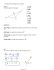More information

### (3) Measurements-2-P2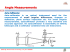More information

### Road Weather Forecast using High Resolution Data from the Danish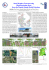More information

### Chapter 4 Angular Measurement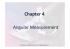More information

### Loupe Optics and Frame Directions for Use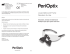More information

### from iaeng.org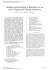More information

### Brunton Omni-Slope Instruction Manual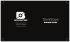More information

### RANCANGAN PELAJARAN TAHUNAN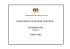More information

### Layouts - Radical Bowling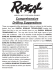More information

### numbers and space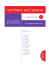More information